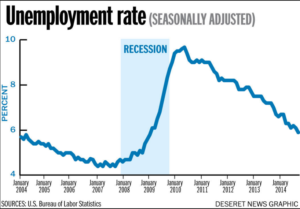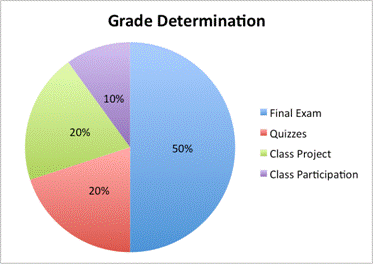## 0.4 – Percent Review

### Learning Objectives

• (0.4.1) – Finding percent of a whole
• (0.4.2) – Solve equations containing percents
• (0.4.3) – Solve percent change and interest problems
• Read and interpret data from pie charts as percents

# (0.4.1) – Finding percent of a whole

Percents are the ratio of a number and 100. Percents are used in many different applications. Percents are used widely to describe how something changed. For example, you may have heard that the amount of rainfall this month had decreased by 12% from last year, or that the number of jobless claims has increase by 5% this quarter over last quarter.Unemployment rate as percent by year between 2004 and 2014.

We regularly use this kind of language to quickly describe how much something increased or decreased over time or between significant events.

Before we dissect the methods for finding percent change of a quantity, let’s learn the basics of finding percent of a whole.

For example, if we knew a gas tank held 14 gallons, and wanted to know how many gallons were in $\frac{1}{4}$ of a tank, we would find $\frac{1}{4}$ of 14 gallons by multiplying:

$\frac{1}{4}\,\cdot \,14=\frac{1}{4}\,\cdot \,\frac{14}{1}=\frac{14}{4}=3\frac{2}{4}=3\frac{1}{2}\,\,\,\text{gallons}$

Likewise, if we wanted to find 25% of 14 gallons, we could find this by multiplying, but first we would need to convert the 25% to a decimal:

$25\%\,\,\text{of}\,\,14\,\,\,\text{gallons}=0.25\,\cdot \,14=3.5\,\,\,\text{gallons}$

### Finding a Percent of a Whole

To find a percent of a whole,

• Write the percent as a decimal by moving the decimal two places to the left
• Then multiply the percent by the whole amount

What is 15% of $200? The following video contains an example that is similar to the one above. From the previous example, we can identify three important parts to finding the percent of a whole: • the percent has the percent symbol (%) or the word “percent” • the amount, the amount is part of the whole • and the base, the base is the whole amount The following examples show how to identify the three parts: the percent, the base, and the amount. ### Example Identify the percent, amount, and base in this problem. 30 is 20% of what number? The previous problem states that 30 is a portion of another number. That means 30 is the amount. Note that this problem could be rewritten: 20% of what number is 30? ### Example Identify the percent, amount, and base in this problem. What percent of 30 is 3? ### Example Identify the percent, amount, and base in this problem. What is 60% of 45? The following video provides more examples that describe how to identify the percent, amount, and base in a percent problem. In the next section, you will use the parts of a percent problem to find the percent increase or decrease of a quantity by writing and solving equations. # (0.4.2) – Solve equations containing percents Percent problems can be solved by writing equations. An equation uses an equal sign (=) to show that two mathematical expressions have the same value. Percents are fractions, and just like fractions, when finding a percent (or fraction, or portion) of another amount, you multiply. In the previous section, we identified three important parts to finding the percent of a whole: • the percent, has the percent symbol (%) or the word “percent” • the amount, the amount is part of the whole • and the base, the base is the whole amount Using these parts, we can define equations that will help us answer percent problems. ### The Percent Equation Percent of the Base is the Amount. $\text{Percent}\cdot\text{Base}=\text{Amount}$ In the examples below, the unknown is represented by the letter n. The unknown can be represented by any letter or a box □, question mark, or even a smiley face :) ### Example Write an equation that represents the following problem. 30 is 20% of what number? The following example shows how to use the percent equation to find the base in a percent equation. Once you have an equation, you can solve it and find the unknown value. For example, to solve $20%\cdot{n}=30$ you can divide 30 by 20% to find the unknown: $20\%\cdot{n}=30$ You can solve this by writing the percent as a decimal or fraction and then dividing. $20\%\cdot{n}=30$ $n=30\div20\%=30\div0.20=150$ ### Example What percent of 72 is 9? In the following video example, you are shown how to use the percent equation to find the base in a percent problem. You can estimate to see if the answer is reasonable. Use 10% and 20%, numbers close to 12.5%, to see if they get you close to the answer. 10% of 72 = 0.1 · 72 = 7.2 20% of 72 = 0.2 · 72 = 14.4 Notice that 9 is between 7.2 and 14.4, so 12.5% is reasonable since it is between 10% and 20%. ### Example What is 110% of 24? The video that follows shows how top use the percent equation to find the amount in a percent equation. # (0.4.3) – Solve percent change and interest problems Percents have a wide variety of applications to everyday life, showing up regularly in taxes, discounts, markups, and interest rates. We will look at several examples of how to use percent to calculate markups, discounts, and interest earned or owed. ### Example Jeff has a coupon at the Guitar Store for 15% off any purchase of$100 or more. He wants to buy a used guitar that has a price tag of $220 on it. Jeff wonders how much money the coupon will take off of the$220 original price.

The example video that follows shows how to use the percent equation to find the amount of a discount from the price of a phone.

You can estimate to see if the answer is reasonable. Since 15% is half way between 10% and 20%, find these numbers.

$\begin{array}{c}10\%\,\,\text{of}\,\,220=0.1\cdot220=22\\20\%\,\,\text{of}\,\,220=0.2\cdot220=44\end{array}$

The answer, 33, is between 22 and 44. So $33 seems reasonable. There are many other situations that involve percents. Below are just a few. ### Example Evelyn bought some books at the local bookstore. Her total bill was$31.50, which included 5% tax. How much did the books cost before tax?

In the following video example, you will be shown how to find a price before tax using the percent equation.

### Example

Susana worked 20 hours at her job last week. This week, she worked 35 hours. In terms of a percent, how much more did she work this week than last week?

The video that follows explains how to use the percent equation to determine the percent increase of a given amount.

### Read and interpret data from pie charts as percents

Circle graphs, or pie charts, represent data as sections of the circle (or “pieces of the pie”), corresponding to their percentage of the whole. Circle graphs are often used to show how a whole set of data is broken down into individual components.

Here’s an example. At the beginning of a semester, a teacher talks about how she will determine student grades. She says, “Half your grade will be based on the final exam and 20% will be determined by quizzes. A class project will also be worth 20% and class participation will count for 10%.” In addition to telling the class this information, she could also create a circle graph.This graph is useful because it relates each part—the final exam, the quizzes, the class project, and the class participation—to the whole.

### Example

If the total number of points possible in the class is 500, how many points is the final exam worth?

In the following video, an example of using a pie chart to determine a percent of a whole is shown.